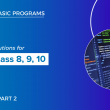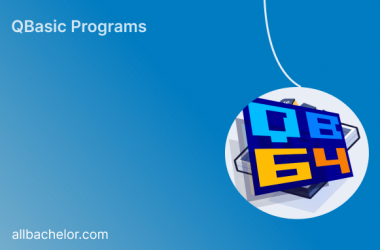# Qbasic programs with solutions(part 1) for class 8, class 9 and class 10

Here, I have listed some of the qbasic programs. We also have mobile app where you can view all the qbasic programs. For better experience I highy recommend you to use our mobile app

## 1. Write a program in qbasicto display volume of sphere.

Solution:

``````REM PROGRAM TO DISPLAY VOLUME OF SPHERE.
CLS
V = (4 / 3) * 3.14 * R ^ 3
PRINT “VOLUME OF SPHERE ”;V
END``````

## 2. Write a program in qbasicto display area of 4 walls

Solution:

``````REM PROGRAM TO DISPLAY AREA OF 4 WALLS
CLS
INPUT “ENTER LENGTH”; L
INPUT “ENTER HEIGHT”; H
A = 2 * H * (L + B)
PRINT “AREA OF FOUR WALLS”; A
END``````

## 3. Write a program in qbasicto display total surface area and volume of cuboid.

Solution:

``````REM PROGRAM TO DISPLAY TOTAL SURFACE AREA AND VOLUME OF CUBOID
CLS
INPUT “ENTER LENGTH OF CUBOID”; L
INPUT “ENTER BREADTH OF CUBOID”; B
INPUT “ENTER HEIGHT OF CUBOID”; H
A= 2 * (L * B + B * H + H * L)
V = L * B * H
PRINT “TOTAL SURFACE AREA OF CUBOID “; A
PRINT “VOLUME OF CUBOID ”; V
END ``````

## 4. Write a program in qbasicto display the total surface area of the cube.

Solution:

``````REM PROGRAM TO DISPLAY TOTAL SURFACE AREA OF CUBE
CLS
INPUT “ENTER SIDE OF A CUBE”; L
T= 6 * L ^ 2
PRINT “TOTAL SURFACE AREA OF CUBE“; T
END ``````

## 5. Write a program in qbasicto display area of rectangle

Solution:

``````REM PROGRAM TO DISPLAY AREA OF RECTANGLE
CLS
INPUT “ENTER LENGTH”; L
A = L * B
PRINT “AREA OF RECTANGLE ”; A
END ``````

## 6. Write a program in qbasicto display the circumference of a circle.

Solution:

``````REM PROGRAM TO DISPLAY CIRCUMFERENCE OF CIRCLE
CLS
C = 2 * 3.14 * R
PRINT “CIRCUMFERENCE OF CIRCLE ”; A
END``````

## 7. Write a program in qbasicto display area of triangle when three sides are given.

Solution:

``````REM PROGRAM TO DISPLAY AREA OF TRIANGLE WHEN THREE SIDES ARE GIVEN
CLS
INPUT “ENTER VALUE FOR FIRST SIDE ”; A
INPUT “ENTER VALUE FOR SECOND SIDE ”; B
INPUT “ENTER VALUE FOR THIRD SIDE ”; C
S = (A + B + C) / 2
AR = (S * (S – A) * (S – B) * (S – C)) ^ (1 / 2)
PRINT “AREA OF TRIANGLE “; AR
END ``````

## 8. Write a program in qbasicto display area and circumference of circle.

Solution:

``````REM PROGRAM TO DISPLAY AREA AND CIRCUMFERENCE OF CIRCLE.
CLS
A= 3.14 * R ^ 2
C = 2 * 3.14 * R
PRINT “AREA OF CIRCLE “; A
PRINT “CIRCUMFERENCE OF CIRCLE ”; C
END
﻿``````

## 9.  Write a program in qbasicto ask distance in kilometer and convert into miles.

Solution:

``````REM PROGRAM TO CONVERT KILOMETER TO MILES
CLS
INPUT”ENTER DISTANCE IN KILOMETER”; K
M = K * 0.62
PRINT “DISTANCE IN MILES=”; M
END ``````

## 10  Write a program in qbasicto input distance in meter and convert into kilometer.

Solution:

``````REM PROGRAM TO CONVERT METER TO KILOMETER
CLS
INPUT "ENTER DISTANCE IN METER"; M
K = M \\1000
PRINT K; "KILOMETER";
END ``````

## 11  Write a program in qbasic to calculate potential energy of body. [PE=MGH where G=9.8]

Solution:

``````REM CALCULATE POTENTIAL ENERGY OF BODY
CLS
INPUT “ ENTER MASS”; M
INPUT “ENTER HEIGHT”; H
P = M * 9.8 * H
PRINT “POTENTIAL ENERGY OF BODY”; P
END ``````

## 12  Write a program in qbasic to display perimeter of rectangle.

Solution:

``````REM PROGRAM TO DISPLAY PERIMETER OF RECTANGLE
CLS
INPUT “ENTER LENGTH”; L
P = 2 * (L + B)
PRINT “PERIMETER OF RECTANGLE ”; P
END ``````

## 13  Write a program in qbasic to input principal , rate,time and display simple interest and total amount.

Solution:

``````REM PROGRAM TO DISPLAY SIMPLE INTEREST AND TOTAL AMOUNT
CLS
INPUT “ENTER PRINCIPAL”; P
INPUT “ENTER TIME”; T
INPUT “ENTER RATE”;R
I = P* T * R / 100
A = P + I
PRINT “SIMPLE INTEREST=”;I
PRINT “TOTAL AMOUNT=”; A
END ``````

## 14  Write a program in qbasicto ask in kilogram and convert into grams.

Solution:

``````REM PROGRAM TO CONVERT VALUE IN KILOGRAM TO GRAMS
CLS
INPUT”ENTER VALUE IN KILOGRAM”; K
G = K * 1000
PRINT “VALUE IN GRAMS=”; G
END ``````

## 15. Write a program in qbasicto display total surface area and volume of sphere.

Solution:

``````REM PROGRAM TO DISPLAY TOTAL SURFACE AREA AND VOLUME OF SPHERE.
CLS
A= 4 * 3.14 * R ^ 2
V = (4 / 3) * 3.14 * R ^ 3
PRINT “TOTAL SURFACE AREA OF SPHERE “; A
PRINT “VOLUME OF SPHERE ”; V
END ``````

## 16  Write a program in qbasicto display total surface area of cylinder.

Solution:

``````REM PROGRAM TO DISPLAY TOTAL SURFACE AREA OF CYLINDER
CLS
INPUT “ENTER HEIGHT”; H
A= 2 * 3.14 * R * (R + H)
PRINT “TOTAL SURFACE AREA OF CYLINDER “; A
END ``````

## 17  Write a program in qbasicto display total surface area of cuboid / box.

Solution:

``````REM PROGRAM TO DISPLAY TOTAL SURFACE AREA OF CUBOID / BOX
CLS
INPUT “ENTER LENGTH”;L
INPUT “ENTER HEIGHT”;H
T= 2 * (L * B + B * H + H * L)
PRINT “TOTAL SURFACE AREA OF CUBOID“;T
END ``````

## 18  Write a program in qbasicto display area and perimeter of rectangle.

Solution:

``````REM PROGRAM TO DISPLAY AREA AND PERIMETER OF RECTANGLE
CLS
INPUT “ENTER LENGTH”; L
A= L * B
P = 2 * (L + B)
PRINT “AREA OF RECTANGLE “; A
PRINT “PERIMETER OF RECTANGLE ”; P
END ``````

## 19  Write a program in qbasic to display total surface area of sphere.

Solution:

``````REM PROGRAM TO DISPLAY TOTAL SURFACE AREA OF SPHERE.
CLS
A= 4 * 3.14 * R ^ 2
PRINT “TOTAL SURFACE AREA OF SPHERE “; A
END ``````

## 20  Write a program in qbasic to calculate distance.[S=UT+1/2(AT2)]

Solution:

``````REM CALCULATE DISTANCE
CLS
INPUT “ENTER INITIAL VELOCITY”; U
INPUT “ENTER TIME”; T
INPUT “ENTER ACCELARATION”; A
S = U * T + 1 / 2 * A * T ^ 2
PRINT “DISTANCE TRAVELLED = “; S
END ``````

You can view other Qbasic programs in 2nd part of this post

1.stainless steel kitchen basin says:

It is actually a nice and helpful piece of information.
simply shared this useful info with us.
Please keep us up to date like this.
Thanks for sharing.

2.Sm Harber says:

I go to see day-to-day a few web sites and blogs to read articles,
except this web
site gives feature based
writing.

3.Stephania Lipinski says:

wonderful as well as amazing blog site. I really want to thank
you, for giving us much better info.

##### Next Post## Qbasic programs solutions(part 2) for class 8, class 9 and class 10

##### Related Posts## Top QBASIC Tips and Tricks for Efficient Coding

Introduction QBASIC, the Microsoft-developed programming language from the 1990s, may have a nostalgic charm, but it can still…## QBASIC and Education: Learning from the Past

Introduction In today’s rapidly evolving technological landscape, integrating an older programming language like QBASIC into education may seem…## Debugging in QBASIC

Debugging is a crucial skill for programmers, even for those using QBASIC, a popular language for beginners. As…